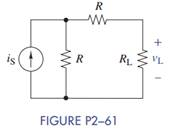Chat Now

# Use current division in Figure P2−61 to find an expression for v L in terms of R, R L , and i...

### Use current division in Figure P2−61 to find an expression for v L in terms of R, R L , and i...

Use current division in Figure P2−61 to find an expression for vL in terms of R, RL, and iS.manish jayant 28-Jul-2020Get solution